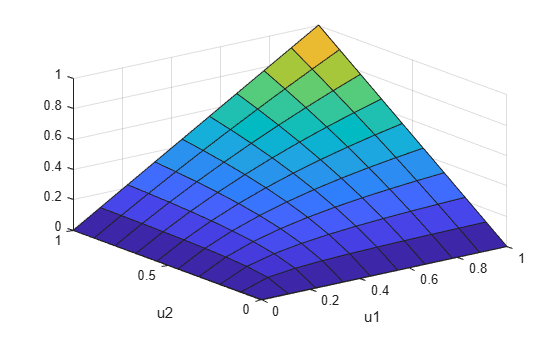copulacdf

Copula cumulative distribution function

Description

y = copulacdf('Gaussian',u,rho) returns the cumulative probability of the Gaussian copula, with linear correlation parameters rho evaluated at the points in u.

y = copulacdf('t',u,rho,nu) returns the cumulative probability of the t copula, with linear correlation parameters, rho, and degrees of freedom parameter nu evaluated at the points in u.

example

y = copulacdf(family,u,alpha) returns the cumulative probability of the bivariate Archimedean copula of the type specified by family, with scalar parameter alpha evaluated at the points in u.

Examples

collapse all

Define two 10-by-10 matrices containing the values at which to compute the cdf.

u = linspace(0,1,10);
[u1,u2] = meshgrid(u,u);

Compute the cdf of a Clayton copula that has an alpha parameter equal to 1, at the values in u.

y = copulacdf('Clayton',[u1(:),u2(:)],1);

Plot the cdf as a surface, and label the axes.

surf(u1,u2,reshape(y,10,10))
xlabel('u1')
ylabel('u2')Input Arguments

collapse all

Values at which to evaluate the cdf, specified as a matrix of scalar values in the range [0,1]. If u is an n-by-p matrix, then its values represent n points in the p-dimensional unit hypercube. If u is an n-by-2 matrix, then its values represent n points in the unit square.

If you specify a bivariate Archimedean copula type ('Clayton', 'Frank', or 'Gumbel'), then u must be an n-by-2 matrix.

Data Types: single | double

Linear correlation parameters for the copula, specified as a scalar value or matrix of scalar values.

• If u is an n-by-p matrix, then rho is a p-by-p correlation matrix.

• If u is an n-by-2 matrix, then rho can be a scalar correlation coefficient.

Data Types: single | double

Degrees of freedom for the t copula, specified as a positive integer value.

Data Types: single | double

Bivariate Archimedean copula family, specified as one of the following.

 'Clayton' Clayton copula 'Frank' Frank copula 'Gumbel' Gumbel copula

Bivariate Archimedean copula parameter, specified as a scalar value. Permitted values for alpha depend on the specified copula family.

Copula FamilyPermitted Alpha Values
'Clayton'[0,∞)
'Frank'(-∞,∞)
'Gumbel'[1,∞)

Data Types: single | double

Output Arguments

collapse all

Cumulative distribution function of the copula, evaluated at the points in u, returned as a vector of scalar values.